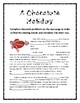# Valentine's Day Math Mystery Stories (Common Core Aligned!)Subject
Resource Type
File Type

PDF

(16 MB|20 pages)
Standards
• Product Description
• StandardsNEW

These Valentine's Day Math Mystery Stories include three short texts about Valentine's Day. These texts include 15 missing words. In order to figure out the missing words students need to solve 15 math problems. The first text has easier math problems, while the second text has medium-level math problems and the third text has harder math problems. All the math problems align with the Common Core State Standards.

This is a great, short activity that involves reading, history and math!

Please let me know if you see any mistakes or have any suggestions.

Thank you!

Feel free to check out my other Math Mystery Stories at the links below...

Labor Day Math Mystery Stories

Patriot Day Math Mystery Stories

Columbus Day Math Mystery Stories

Veterans Day Math Mystery Stories

Martin Luther King Jr. Day Math Mystery Stories

Groundhog Day Math Mystery Stories

Presidents’ Day Math Mystery Stories

April Fool’s Day Math Mystery Stories

Cinco de Mayo Math Mystery Stories

Memorial Day Math Mystery Stories

Flag Day Math Mystery Stories

Apply and extend previous understandings of multiplication to multiply a fraction or whole number by a fraction.
Add and subtract fractions with unlike denominators (including mixed numbers) by replacing given fractions with equivalent fractions in such a way as to produce an equivalent sum or difference of fractions with like denominators. For example, 2/3 + 5/4 = 8/12 + 15/12 = 23/12. (In general, 𝘢/𝘣 + 𝘤/𝘥 = (𝘢𝘥 + 𝘣𝘤)/𝘣𝘥.)
Apply and extend previous understandings of multiplication to multiply a fraction by a whole number.
Add and subtract mixed numbers with like denominators, e.g., by replacing each mixed number with an equivalent fraction, and/or by using properties of operations and the relationship between addition and subtraction.
Fluently multiply and divide within 100, using strategies such as the relationship between multiplication and division (e.g., knowing that 8 × 5 = 40, one knows 40 ÷ 5 = 8) or properties of operations. By the end of Grade 3, know from memory all products of two one-digit numbers.
Total Pages
20 pages
Included
Teaching Duration
N/A
Report this Resource to TpT
Reported resources will be reviewed by our team. Report this resource to let us know if this resource violates TpT’s content guidelines.